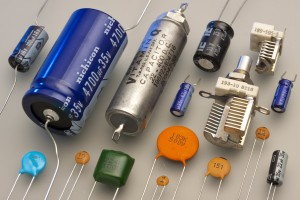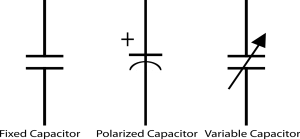# Capacitors: Symbols and Markings

Capacitor is a two terminal passive device used to store energy in the form of electric charge. It is comprised of two parallel plates which are separated from each other either by air or by some other insulating device like paper, mica, ceramic etc. In this tutorial,we put some shadow on the capacitance of the capacitor, Capacitor symbol and capacitor markings etc.## Capacitance of capacitor

The Capacitor is made up of two conductors separated by an insulator i.e. dielectric. The dielectric can be of different types, you can use any of the dielectric material between the plates of the capacitor as per your need. The amount of electrical energy stored in the capacitor is known as its capacitance. The Capacitance of a capacitor is directly proportional to the capacity of the capacitor for storing charge e.g. the bigger the tank, the more water it can store similarly the bigger the capacitance, the more charge it can store. In three ways you can increase the capacitance of the capacitor are:

1. By increasing the size of the plates.
2. By decreasing the distance between the plates.
3. Make dielectric as good as an insulator.

## Capacitor symbols and units

#### (i) Capacitor Symbol:

Its symbol consists of two parallel lines separated from each other i.e. Flat, curved or an arrow passes through it. The flat line indicates that the capacitor is non-polarized, the curved line indicates that the capacitor is polarized and arrow type indicates that it is of a variable type.#### (ii) Capacitor Unit:

Capacitance of capacitor is measured in Farads symbolised as F. It is defined as being that a capacitor has the capacitance of one Farad when one coulomb of electric charge is stored in the conductor on the application of one volt potential difference. It has no negative units, it is always positive. The charge stored in a capacitor is given by:

Q = CV

Where Q : charge stored by the capacitor

C : Capacitance value of the capacitor

V : Voltage applied across the capacitor

We can connect capacitor either in series or in parallel as per requirement, one thing is to remember is that the formula is different for calculation in series or parallel.

If we connected capacitor in series then the capacitance formula is:

1/Cs = 1/C1 + 1/C2 + 1/C3 +… + 1/Cn

If we connected capacitor in parallel then the capacitance formula is:

Cp = C1 + C2 + C3 +… + Cn

### Capacitor markings:

Capacitors are marked in different ways depends upon its colour code, voltage code, Tolerance code and temperature coefficient etc. Here we explain you meaning and values of all such codes marked on different Types of capacitors.

(i) Colour code: Different schemes are used for different types of capacitors. Nowadays this type of capacitor marking is using less these days but it is available on some older components.

(ii) Tolerance code: Some capacitors have tolerance code depends upon its dielectric material. Following tolerance rating is given below.

Capacitor Tolerance Code and Capacitance Values

 Alphalet on Capacitor Capacitor Tolerance Z +80%, -20% M +- 20% K +- 10% J +- 5% G +- 2% F +- 1% D +- 0.5% C +- 0.25% B +- 0.1%

(iii) Temperature coefficient codes: On several capacitors marking or code indicates the temperature coefficient of the capacitor. All these codes are standardized by EIA (Electronics Industries Alliance), these codes are typically used for ceramic and film type capacitors.

Capacitor Temperature Coefficient codes

 Electronic Industries Alliance (EIA) Industry Temperature Coefficents C0G NP0 0 S1G N033 -33 U1G N075 -75 P2G N150 -150 S2H N330 -330 U2J N750 -750 P3K N1500 -1500

(iv) Capacitor working voltage codes: Working voltage is the key parameter of any electronic component. Sometimes capacitors are of smaller size and it is not possible to write whole code over it, so for this purpose we write only one character over it which designates specific voltage values. Below we are providing a table which indicates the specific voltage values of the capacitor.

Capacitor Working Voltage Codes

 Letter Voltage EIA capacitor voltage codes e 2.5 0e = 2.5VDC G 4 0G = 4.0VDC J 63 0J = 6.3VDC , 1J = 63VDC A 10 1A = 10VDC, 2A = 100VDC C 16 1C = 16VDC, 2C= 160VDC D 20 2D = 200VDC E 25 1E = 25VDC , 2E = 250VDC V 35 2V = 350VDC H 50 1H = 50VDC

(v) Number code:
Most capacitors have a number printed on their body indicates their electrical properties. Capacitors like electrolytic are larger in size usually display the actual capacitance together with the unit like 120 µF while capacitors like ceramic are smaller in size use short notations of three numeric digit and letter where digit indicates the capacitance value in pF and letter indicates tolerance. For example, let’s consider text 343M 220V available on the body of the capacitor. It designates 34 x 103 pF = 34 nF (±20%) with the working voltage of 220V. To prevent the risk of breaking down the dielectric layer always use highest working voltage.

After knowing all such things regarding its symbols and markings, you can now proceed further and gather some information about its Construction and working.

#### Applications of capacitors:

Capacitors are widely used in the electronic industries. Following of its application are:

1. It is used in coupling of two stages of a circuit.
2. Used in filter networks.
3. Used for smoothing the output of the power supply circuits.
4. Used for delay application like in 555 timer IC controlling the charging and discharging.
5. It is used for Phase alteration.
6. In camera flash circuits, it stores charges.

Ajay Kumar

Hi readers, Ajay Kumar is graduated in Electronics and Communication Engineering is responsible for running this website. With the knowledge, he has gained, he tries to provide the readers as much of it as he can.

## 2 thoughts on “Capacitors: Symbols and Markings”

1. Samuel says:

Hi
Iam sam, I would like to know the difference between audio purpose and general purpose capacitors which have the same values.

This site uses Akismet to reduce spam. Learn how your comment data is processed.